## Tamilnadu Samacheer Kalvi 6th Maths Solutions Term 2 Chapter 5 Information Processing Additional Questions

Question 1.
Draw tree diagrams for the following questions:
(i) The number of books sold in a book fair is as follows. First day 1,82,192, Second day 1,28,194, Third day 80,520 fourth day 92,004 and the fifth day 50,020. Find the total number of the book sold.
(ii) A water purification project cost 1,82,71,000. The machinery was bought for ₹ 69,12,000. What is the amount needed to complete the project?
(iii) The number of flowers needed to arrange in a flower pot is 62. Find the number of flowers needed to arrange in 55 pots?
(iv) If the total scholarship money sanctioned for 50 students are ₹ 62,000. Find the amount that each student can get?
Solution: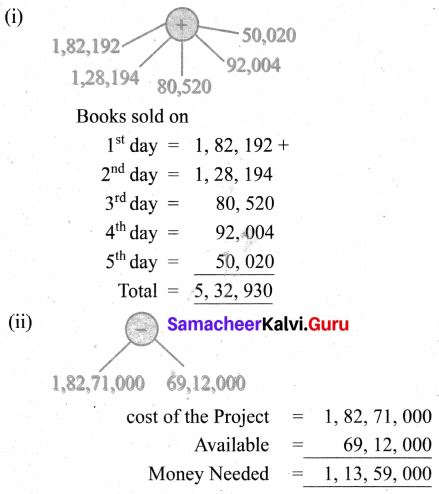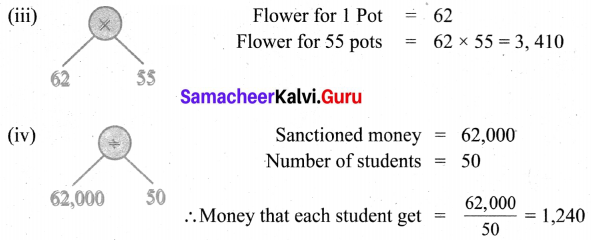Question 2.
Convert into tree diagrams
(i) (10 × 5) + (2 × 16)
(ii) (5 × 3) – (8 × 6) + 9
(iii) [9 + (3 × 2)] – [(6 × 4) + 5]
(iv) [(4 – 1) × 16] + [(16 + 9) × 3]
(v) {[(10 × 6) + 5] × [ 4 + (3 – 2)]} ÷ [4 × (2 + 9)]
(vi) 4 + [8 × 6 + {(4 × 3) – (10 ÷ 4)}]
Solution: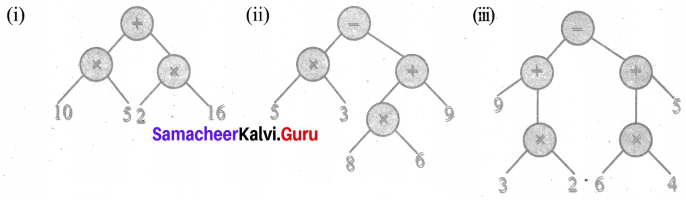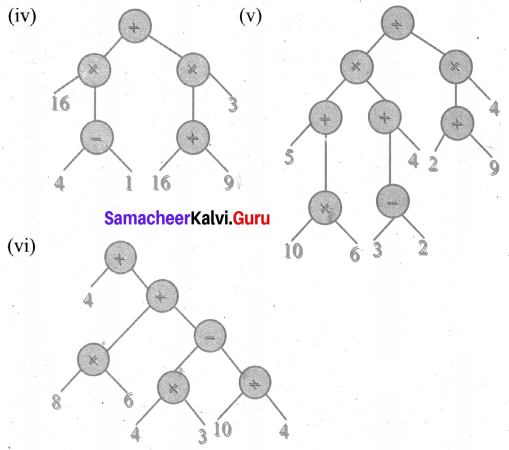Question 3.
Convert the following tree diagrams into numerical expressions.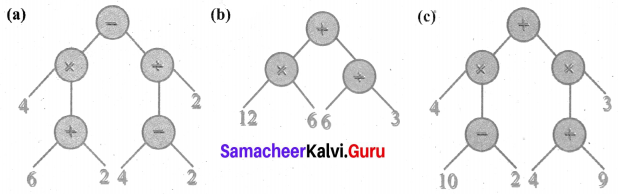Solution:
(a) [4 × (6 + 2)] – [(4 – 2) ÷ 2]
(b) (12 × 6) + (6 ÷ 3)
(c) [4 × (10 – 2)] + [(4 + 9) × 3]